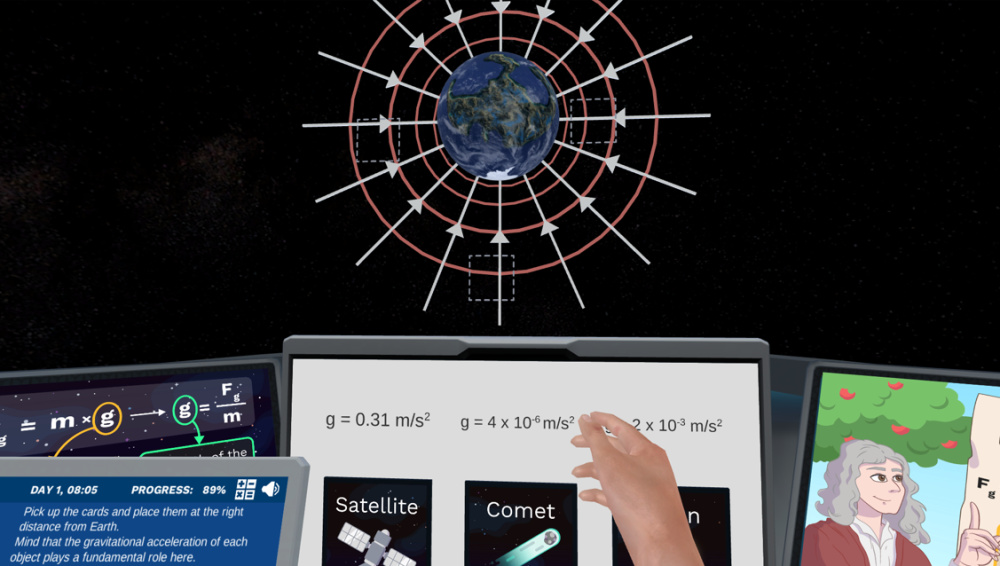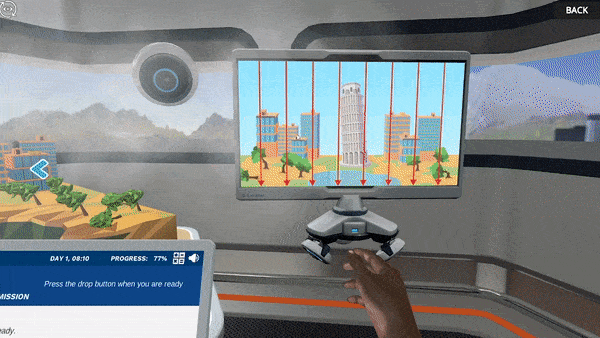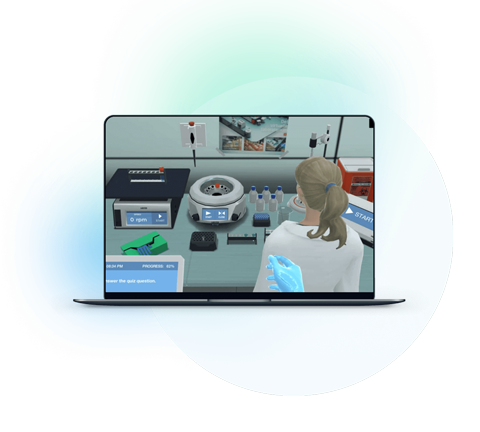Blog

# 5 Ways to Get Students Energized About Gravitational Field

Teaching with Labster
November 2, 2022

Gravity is an invisible force that we experience in our everyday lives. It keeps us on the ground and prevents us from flying off into space. It’s also responsible for the tides in the oceans.

We experience the force of gravity everywhere on earth. However, the magnitude of this force decreases as we move away. A region in space around a mass where the force of gravity is exerted is called the gravitational field.

The concept of the gravitational field was introduced by Isaac Newton. In his law of universal gravitation, Newton showed that the force between two masses is proportional to the product of their masses and inversely proportional to the square of the distance between them.

Understanding the gravitational field can help students understand the way the universe works. It can help them understand the working of the GPS, how the tides are caused and how celestial objects orbit in space.

It is one of the fundamental concepts in physics, and as an educator, you need to familiarize students with them at the start of your course. However, at times, teaching it can be a little trickier.Image from Labster's lab simulation on the gravitational field,

Continue reading to learn three reasons why students find the concept of the gravitational field tricky and five techniques you can adopt to make it an approachable topic.

## 3 Reasons Why Students Find Gravitational Field Tricky to Understand

Physics is an interesting subject involving the study of the nature and properties of matter and energy. However, some topics can be difficult to understand, especially if students are not interested in the subject. One such topic is the gravitational field. Here are three reasons why students find it tricky:

### 1. Gravitational Field is an Abstract Concept

One of the main reasons students find it hard to understand the concept of the gravitational field is that it is abstract. It is not something we can touch or see; we only observe its effects on objects.

### 2. Some Terms Confuse Students

Some of the terms used to describe the gravitational field can be confusing for students. For example, the term "field lines" can be confusing because it makes it sound like the gravitational field is something that has a physical structure. However, they are just imaginary lines used to define the scope of the gravitational field.

### 3. It’s Complex

The concept of a gravitational field is a bit complex since it involves the interaction of two or more masses, and the force exerted by the gravitational field on objects. This makes it tough for some students to comprehend.

## 5 Ways to Familiarize Students with Gravitational Field

Keeping in mind the reasons why students find the concept of the gravitational field hard to understand, here are five ways to get them excited about it.

### 1. Show Them People Behind Science

To get them excited about the topic, you can narrate some interesting stories about the people who introduced that concept; something that’s important and makes them curious. This will help you grab the student’s attention and you will be able to deliver your content more effectively.

Most of the educators mention Newton’s story of the falling apple and how he gets the idea of gravity. You can narrate that too. However, long before Newton gave that idea, there was another scientist named Galileo who gave a similar concept through his experiment. A legend tells us that Galileo dropped a few weights from the famous leaning tower of PISA and observed that each of the weights, despite their magnitude, accelerated downward with a constant acceleration, which later was called gravitational acceleration.

Two centuries after that Newton gave his law of gravitation, which further explained the concept mathematically. Albert Einstein also gave his theory of relativity, which explained gravity in a more modern way.

### 2. Explain the basic terms first

One of the best strategies is to start with the basic terms. If you directly jump on to the complex terms, it's obvious that most students wouldn't get interested in it since they don't know its background and initial terms.

In this case, you have to make sure that your students understand the basic terms related to the gravitational field before you start teaching them the concept. For instance, explain the concept of mass, weight, gravity and gravitational field, etc. Then, you can move on to the law of universal gravitation.

Mass: The quantity of matter that an object contains

Weight: The force with which gravity pulls on an object

Gravity: The force that attracts objects toward the center of the Earth

Gravitational Field: The space around a mass where its gravitational force is felt.

Acceleration due to Gravity: The acceleration gained by an object when it freefalls under the influence of gravity

Newton’s Law of Universal Gravitation: The force of gravity between two masses is proportional to the product of their masses and inversely proportional to the square of the distance between them.

Explain the differences between mass and weight:

One of the common confusions among students is the difference between mass and weight.  The two concepts are often confused because they are related to each other; the weight of an object is directly proportional to its mass. However, the two concepts are different, and students need to understand the difference between them.

Mass is the quantity of matter that an object contains, and it is measured in kilograms. Weight is the force with which gravity pulls on an object, and it is measured by Newton. Mass is constant, but weight can vary depending on the gravitational pull.

For instance, a person has the same mass on Earth as he does on the Moon, but his weight would be less on the Moon because the gravitational force is weaker there.

### 3. Cite Applications of Gravity

Students may find it challenging to visualize gravity. However, contextualizing the lessons and connecting them to real-world applications can make this subject intriguing.

We are Under the Influence of Gravity

The biggest example you can quote to students is our life on Earth, which is under the influence of strong gravitational force. Gravity is what keeps us on the ground and prevents us from flying off into space. Similarly, it’s gravity that makes things fall when we drop them.

Tides in Oceans

Gravity is also responsible for the tides in the oceans. The moon’s gravitational pull causes the water on Earth to bulge out towards the moon. This results in a high tide on the side of Earth facing the moon and a low tide on the opposite side.

Satellites' Motion

Gravity also affects the trajectory of objects in space. Natural satellites such as the Moon orbit around Earth because of Earth’s gravitational force, which provides them enough centripetal force to continue their circular motion.

Similarly, all artificial satellites, including the GPS satellites that we use daily for navigation, are in orbit around Earth because of gravity.

### 4. Design Experiments where Students Measure Gravity

Since Physics explains physical phenomena, it needs to be explained effectively with practical demonstrations. For that, you need to design experiments where students can measure the gravitational force. For example,

1) Measuring the local acceleration due to gravity: In this experiment, students drop an object from a known height and measure the time it takes to hit the ground. From this data, they can calculate the acceleration of gravity near the Earth’s surface.

2) Measuring the weight of an object: In the experiment, students measure the force required to hold an object stationary using a spring balance. This measurement is equal to the object’s weight.

3) Investigating the relationship between mass and weight: In this experiment, students first measure the mass using a physical balance and then measure the weight using a spring balance. They can then compare the weights to the masses of the objects to investigate the relationship between mass and weight.

4) Determining the acceleration due to gravity from a pendulum: In this experiment, students measure the period of a pendulum as a function of the pendulum’s length. From this data, they calculate the acceleration of gravity.

You can similarly design multiple other interesting experiments; the purpose must be to give them a practical demonstration of how gravity is involved.

### 5. Use Virtual Lab Simulations

One way is physically experimenting with things in pre-designed physics labs. However, all these experiments can be done through virtual labs as well. Through Labster's lab simulations, you can easily explain the gravitational field and let students perform all experiments virtually.

The Labster simulations are very comprehensive and well-designed. They provide an immersive experience to users which facilitates long-term retention of information.

For instance, you can check out our lab simulation on the gravitational field, in which students visually see and experience the gravitational field. They conduct different experiments to measure the force of gravity.Figure from Labster showing how students perform a drop test and note its time.